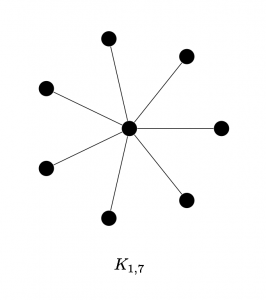# LaTeX/TikZ to draw a star graph $K_{1,n}$

For a diagram for a class this week, I’ve written a LaTeX command to draw star graphs using TikZ. A star graph $K_{1,n}$ is a graph with a single central node, $n$ radial nodes, and $n$ edges connecting the central node to each radial node. I am sharing this here in case it is useful to anyone else.

The result is a command \stargraph, which takes two parameters, the number of radial nodes and the radius in cm of the graph. Here is the definition of \stargraph and its use in a document.

\documentclass{article}
\usepackage{tikz}
\newcommand{\stargraph}{\begin{tikzpicture}
\node[circle,fill=black] at (360:0mm) (center) {};
\foreach \n in {1,...,#1}{
\node[circle,fill=black] at ({\n*360/#1}:#2cm) (n\n) {};
\draw (center)--(n\n);
\node at (0,-#2*1.5) {$K_{1,#1}$}; % delete line to remove label
}
\end{tikzpicture}}

\begin{document}
\begin{center}
\stargraph{7}{2}
\end{center}
\end{document}

Here is the result of making this document:Tags: , ,

•